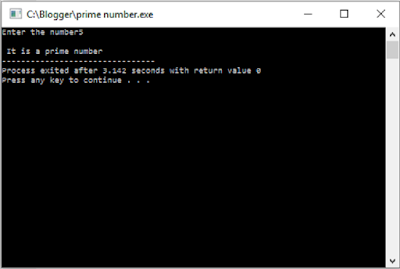## Friday, 29 January 2016

### Write a C program to find if the given number is a prime number or not - Online Rockers

Good Morning viewers,

I am starting my day with this post. Seeing the title above , you may think weird such that how can we feed the logic to a computer to know if the entered number is a prime number or not. But the fact is that it is so simple.

### How will you find if a number is a prime number or not?

Generally speaking , numbers above 1 can only be a prime number or a composite number . Any number which has its factors 1 and itself is called prime number. For example , 7 has its factors 1 and 7 . Hence, it is a prime number.If a number has factors other than 1 and itself then it is called Composite number.

Logically , we know to find a prime number , by checking if the number is divisible by any other number . Now , a doubt may arise in my mind thinking , how can a computer check this,  Don't worry , I am gonna show you how to do this.

Note:In order to compile a C program , you must have a C compiler. You can download it here for absolutely  free,

### Source code:

#include<stdio.h>
int main()
{
int num,i=2;
printf("Enter the number");
scanf("%d",&num);                                             //accept number from user
while(i<=(num-1))                                               //loop to check prime number
{
if(num%i==0)
{
printf("\n It is not a prime number");
break;                                                   //printing the result
}
else
{
i++;
}
}
if(i==num)
{
printf("\n It is a prime number");                   //printing the result
}
return 0;
}

### Output:

#### Sample output 1:#### Sample output 2:### Check out other C programs:

Subscribe to us to get more interesting and useful programs.
Subscribe by entering your email in the follow by email widget at footer.
Share it on social media with your friends.